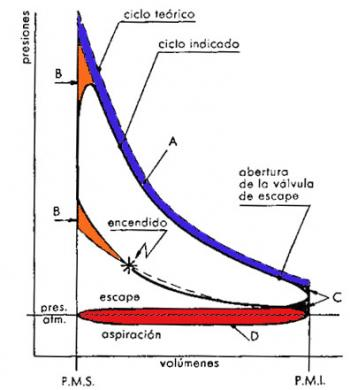# Difference between real and theoretical Otto cyclesBetween the Otto real cycle and the corresponding theoretical Otto there are substantial differences. Some of these differences can be observed when comparing the diagram of the real cycle with the theoretical cycle diagram. The other differences that we are going to analyze refer to the differences between the temperature and pressure values of the two Otto engine cycles.

## Differences in the form of the diagram

The difference in shape of the diagram between a real Otto cycle and a theoretical Otto cycle consists of a different profile in the expansion and compression curves, in the replacement of the rectilinear lines of introduction and subtraction of the heat by curved lines and the rounding of the acute angles. The causes of such differences are based on the following reasons:

• Heat losses
• Non-instantaneous combustion
• Exhaust valve opening time

### Heat losses

In the theoretical cycle, heat loss is considered null. On the other hand, in the real Otto cycle, heat losses are quite sensitive.

One of the characteristics of the thermal engine is that the cylinder is cooled to ensure a good functioning of the piston. The drawback of keeping the cylinder cool is that a certain heat part of the fluid is transmitted to the walls.

The lines of compression and expansion are not, therefore, adibatic, but polytropic, with an exponent n, different from k. As the fluid experiences a loss of heat energy, it is evidently: for expansion, n> k, and for compression.

### Non-instantaneous combustion

In the theoretical cycle, it is assumed that combustion takes place at constant volume; it is, therefore, instantaneous. In the real Otto cycle, on the contrary, the combustion lasts a certain time. If the ignition takes place exactly at the PMS, the combustion will occur while the piston moves away from said point, and the value of the pressure would be lower than expected, with the corresponding loss of useful work.

It is therefore necessary to anticipate the ignition of gasoline so that combustion can take place, for the most part, when the piston is in the vicinity of the PMS This produces a rounding of the theoretical line 2-3 of introduction of thermal energy (heat). This yielding of the curve implies a loss of useful work represented by the area B. However, this loss of work results in a quantity that is much smaller than that which would be had without advancing the ignition.

### Opening time to the exhaust valve

In the theoretical Otto cycle, it is also assumed that the subtraction of heat occurred instantaneously in the PMI. In the real Otto cycle the subtraction of heat takes place at a time when a part of the gases leave the cylinder before the piston reaches the PMI so that its pressure drops close to the value of the external pressure at the beginning of the ejection stroke.

This fact causes a loss of useful work represented by area C, a loss that is, however, smaller than that which would be had without the advance of the opening of the exhaust valve.

## Differences in pressure and temperature values

The causes of the differences in the values of pressure and maximum temperature between the real Otto cycle and the theoretical Otto cycle are the following:

• Increase of specific heats of fluid with temperature
• Dissociation in combustion

### Increase of specific heats of fluid with temperature

As we already know, both the specific heat at constant pressure cp and the corresponding constant volume cv, of a real gas, grow with the temperature, but in such a way that their difference remains constant, that is, cp - cv = AR; consequently, increasing the temperature decreases the value of the relation k = cp / cv. From which it is inferred that the values of the maximum pressure and temperature are always lower than those that would be reached in the case in which the specific heats remained constant when the temperature changed.

This fact is also taken into consideration when tracing the theoretical cycle of air; but, in the real case, the combustion products have specific heats greater than air, and, therefore, the values of pressure and maximum temperature are, in the real cycle, lower than those corresponding to the theoretical cycle. For this reason, surface and thermal performance are diminished.

### Dissociation in combustion

The products of combustion are essentially CO2 and H2O, in addition to other compounds, such as CO, H2 and O2. The dissociation of these products is a reaction that takes place with the absorption of heat energy, the maximum achievable temperature is lower and a certain amount of work is lost. But since the temperature decreases during the expansion, a recession in the dissociation reaction occurs. As a result, a partial re-association with heat development ensues in this phase. The value of the exponent of the expansion polytropics decreases - which should be greater than k due to the heat losses through the walls of the cylinder - and approaches that of the compression polythropic; therefore, a partial recovery of the previously lost work is achieved.

The real cycle of the Otto engine presents, finally, another important difference when compared with the theoretical Otto cycle; during the suction stroke, the pressure in the cylinder is lower than that in the exhaust stroke. Except for specific cases, in the course of the suction, the pressure is lower than the atmospheric pressure, while during the escape it is higher. A negative surface (D, in the figure), corresponding to the lost work, is created in the indicated diagram.

The effort made by the Otto engine to carry out the aspiration and the escape is called pumping work and this, generally, included in the work lost due to friction.

valoración: 3.2 - votos 28

Last review: April 26, 2018